# PSAT Math : How to find the lowest / least common denominator

## Example Questions

### Example Question #1 : How To Find The Lowest / Least Common Denominator

3/5 + 4/7 – 1/3 =

7/9

88/105

4/3

72/89

3/37

88/105

Explanation:

We need to find a common denominator to add and subtract these fractions. Let's do the addition first. The lowest common denominator of 5 and 7 is 5 * 7 = 35, so 3/5 + 4/7 = 21/35 + 20/35 = 41/35.

Now to the subtraction. The lowest common denominator of 35 and 3 is 35 * 3 = 105, so altogether, 3/5 + 4/7 – 1/3 = 41/35 – 1/3 = 123/105 – 35/105 = 88/105. This does not simplify and is therefore the correct answer.

### Example Question #1 : How To Find The Lowest / Least Common Denominator

3/5 + 4/7 – 1/3 =

88/105

7/9

72/89

4/3

3/37

88/105

Explanation:

We need to find a common denominator to add and subtract these fractions. Let's do the addition first. The lowest common denominator of 5 and 7 is 5 * 7 = 35, so 3/5 + 4/7 = 21/35 + 20/35 = 41/35.

Now to the subtraction. The lowest common denominator of 35 and 3 is 35 * 3 = 105, so altogether, 3/5 + 4/7 – 1/3 = 41/35 – 1/3 = 123/105 – 35/105 = 88/105. This does not simplify and is therefore the correct answer.

### Example Question #1 : General Fractions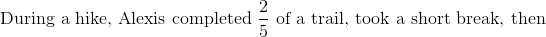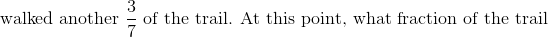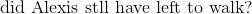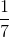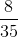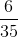Explanation: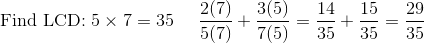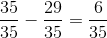### Example Question #3 : General Fractions

Simplify: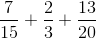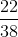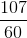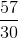Explanation:

First, find the common denominator of the fractions in order to add them together. Looking at the first two fractions, we can see that the common denominator is 15 because 3 times five is 15. We can now change both fractions to have a denominator of 15 so that we can add them together: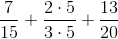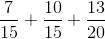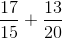Now, find a common denominator for the remaining fractions. This can be done by listing multiples of 15 and 20 and finding the lowest common multiple:

15: 15, 30, 45, 60, 75

20: 20, 40, 60, 80

60 is the lowest common multiple, so it is our least common denominator.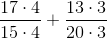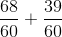### All PSAT Math Resources